# Psuedo Code Pseudo Code Pseudo Fake Code Fancy

• Slides: 29Psuedo CodePseudo Code • Pseudo = Fake • Code = Fancy computer stuff • A way to organize your program before coding it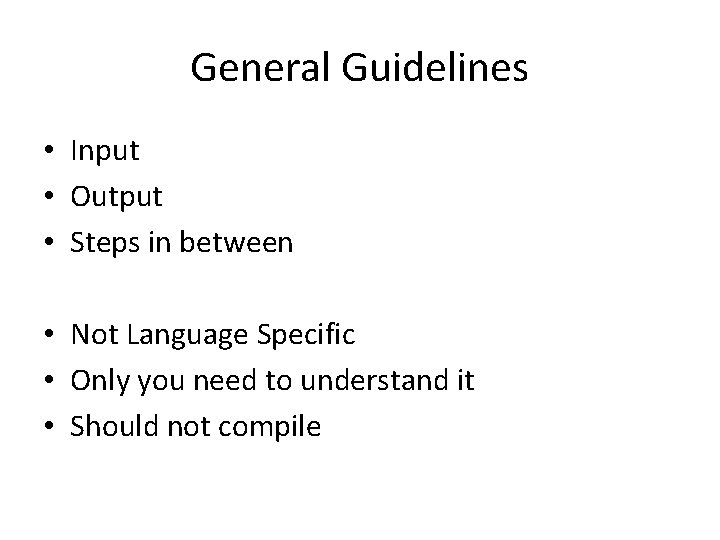General Guidelines • Input • Output • Steps in between • Not Language Specific • Only you need to understand it • Should not compileSteps to Pseudo Coding • Step 1: Outline – Very General, Inputs to outputs – Little computer code used – Short and to the point • Step 2: Actual Pseudo Code – More line by line – Focus on variable storage and functions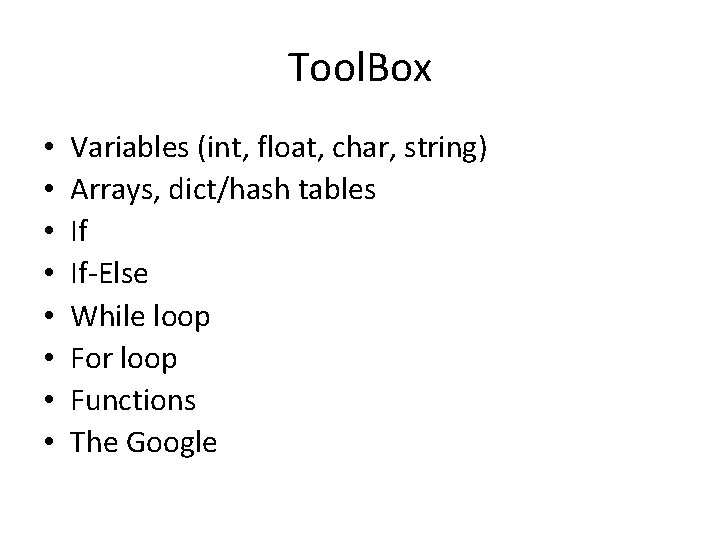Tool. Box • • Variables (int, float, char, string) Arrays, dict/hash tables If If-Else While loop For loop Functions The Google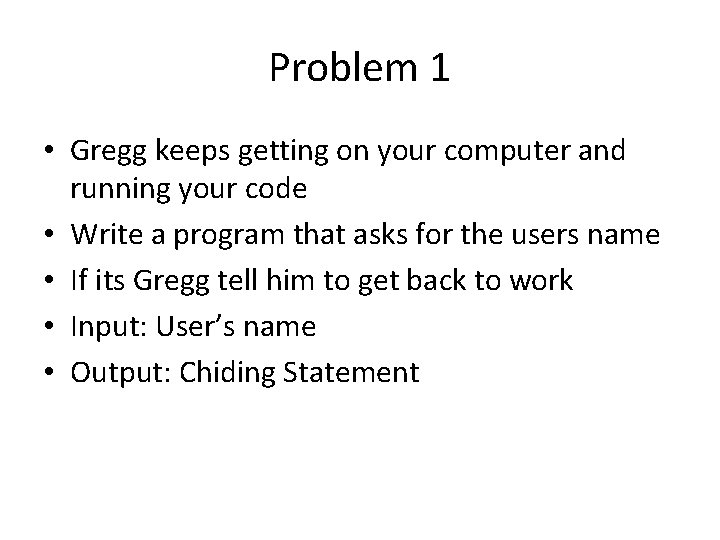Problem 1 • Gregg keeps getting on your computer and running your code • Write a program that asks for the users name • If its Gregg tell him to get back to work • Input: User’s name • Output: Chiding Statement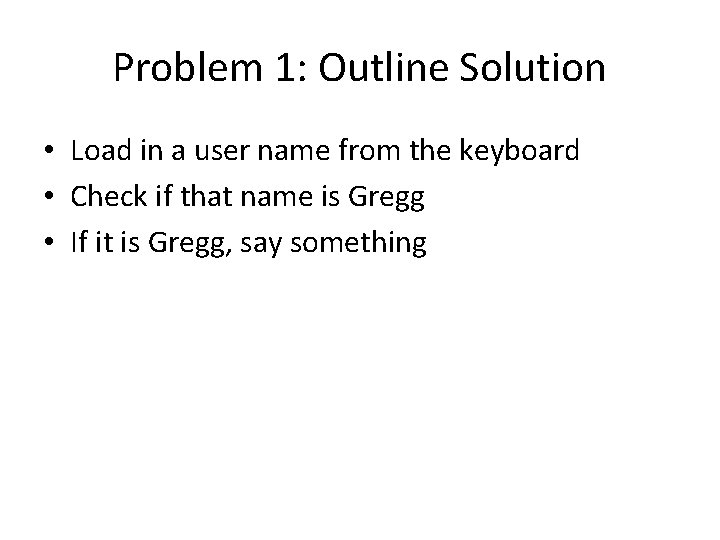Problem 1: Outline Solution • Load in a user name from the keyboard • Check if that name is Gregg • If it is Gregg, say something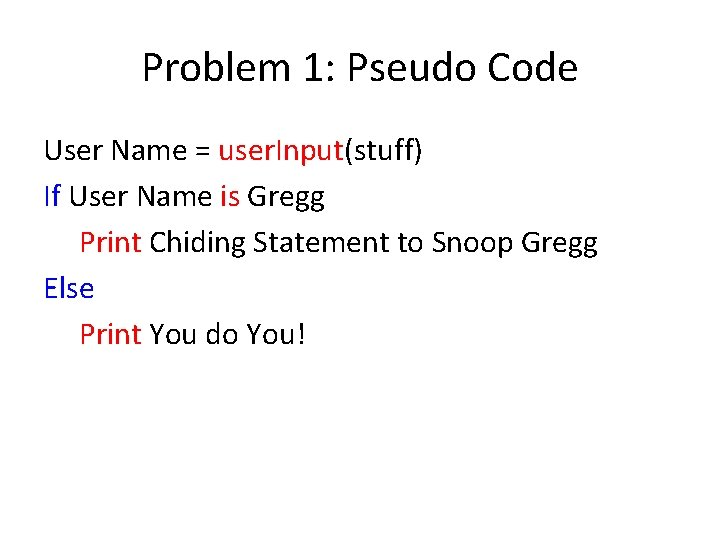Problem 1: Pseudo Code User Name = user. Input(stuff) If User Name is Gregg Print Chiding Statement to Snoop Gregg Else Print You do You!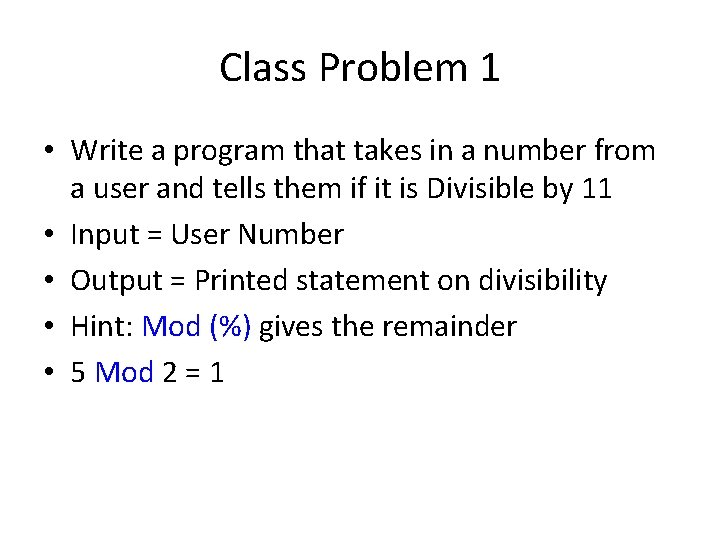Class Problem 1 • Write a program that takes in a number from a user and tells them if it is Divisible by 11 • Input = User Number • Output = Printed statement on divisibility • Hint: Mod (%) gives the remainder • 5 Mod 2 = 1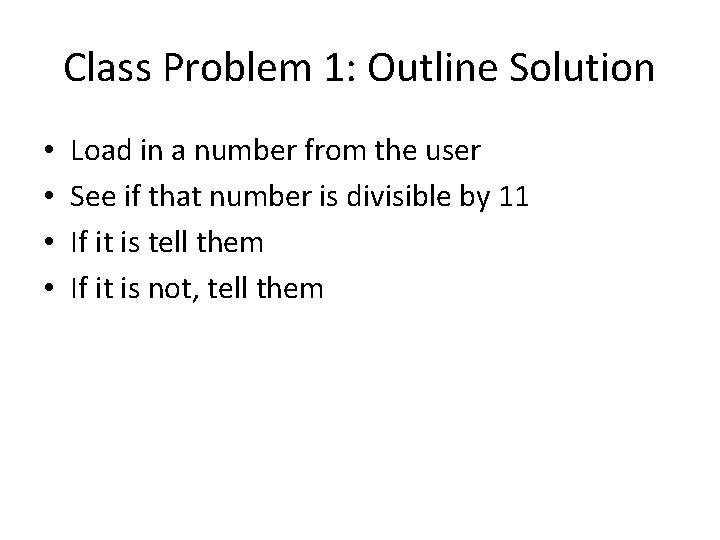Class Problem 1: Outline Solution • • Load in a number from the user See if that number is divisible by 11 If it is tell them If it is not, tell them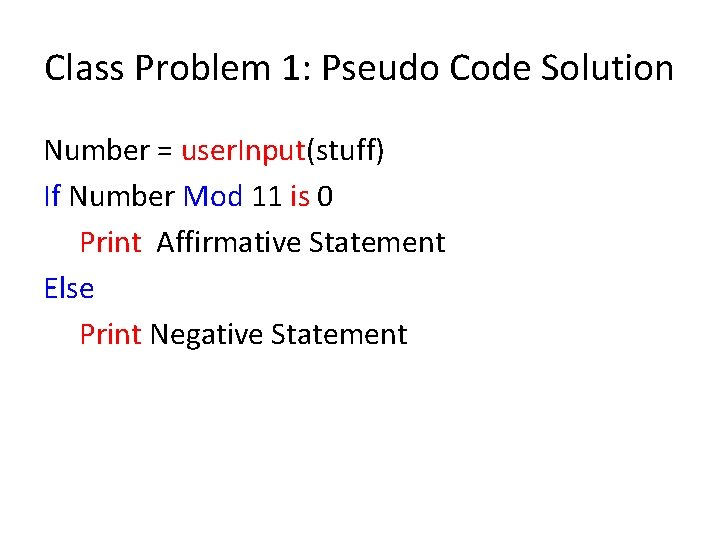Class Problem 1: Pseudo Code Solution Number = user. Input(stuff) If Number Mod 11 is 0 Print Affirmative Statement Else Print Negative StatementProblem 2 • Write a program that takes in a number from a user and tells them if it is Divisible by 11 • But keep asking until the user enters the number 0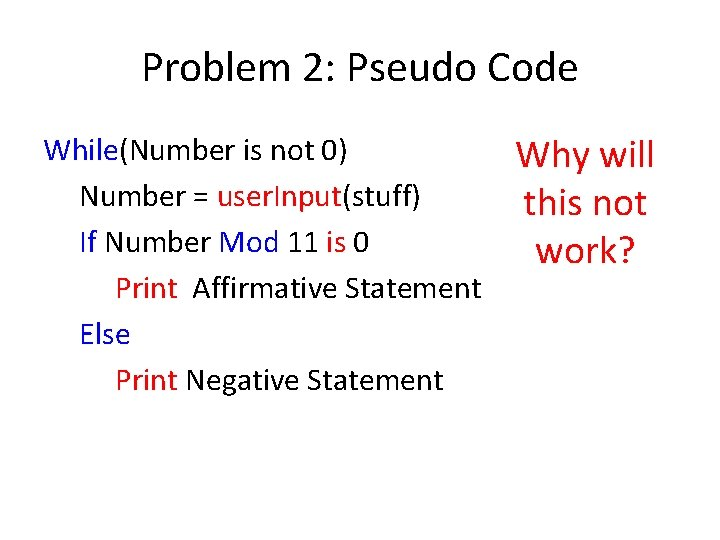Problem 2: Pseudo Code While(Number is not 0) Number = user. Input(stuff) If Number Mod 11 is 0 Print Affirmative Statement Else Print Negative Statement Why will this not work?Class Problem 2: Pseudo Code Number = not 0 While(Number is not 0) Number = user. Input(stuff) If Number Mod 11 is 0 Print Affirmative Statement Else Print Negative Statement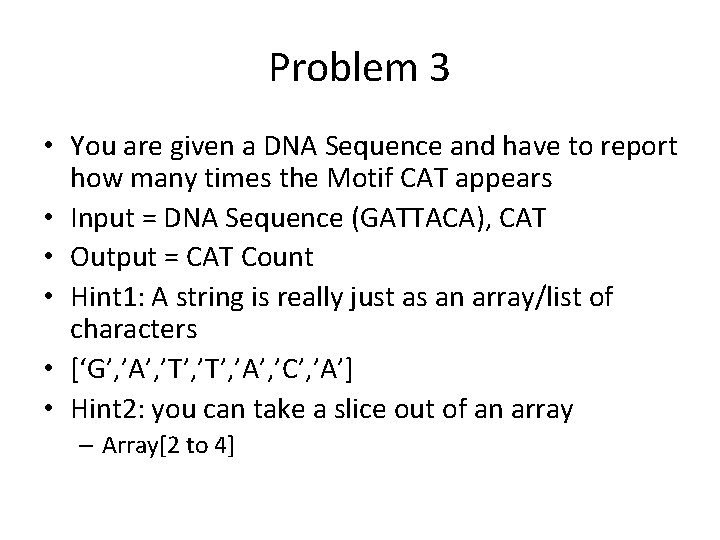Problem 3 • You are given a DNA Sequence and have to report how many times the Motif CAT appears • Input = DNA Sequence (GATTACA), CAT • Output = CAT Count • Hint 1: A string is really just as an array/list of characters • [‘G’, ’A’, ’T’, ’A’, ’C’, ’A’] • Hint 2: you can take a slice out of an array – Array[2 to 4]Problem 3: Outline • Load DNA Sequence and CAT into variables • make a motif counter • Iterate through DNA sequence – Pull out sets of three letters and compare to CAT – If we find CAT increment the counter • Report the final countVisual Representation • G A T T A C A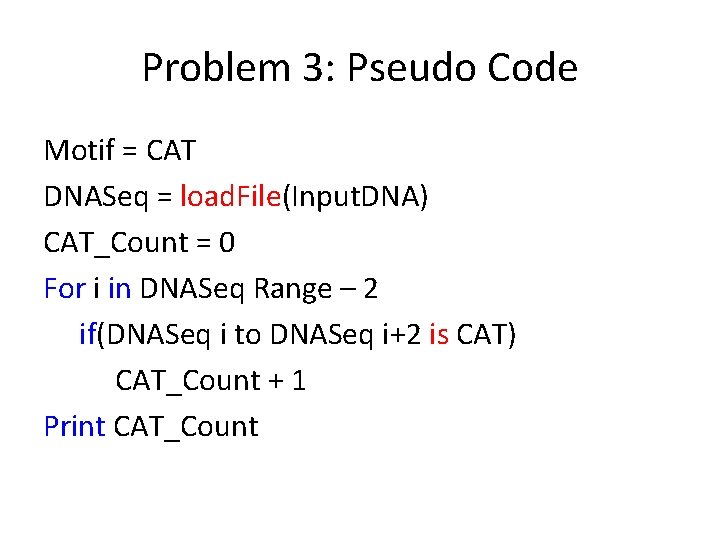Problem 3: Pseudo Code Motif = CAT DNASeq = load. File(Input. DNA) CAT_Count = 0 For i in DNASeq Range – 2 if(DNASeq i to DNASeq i+2 is CAT) CAT_Count + 1 Print CAT_Count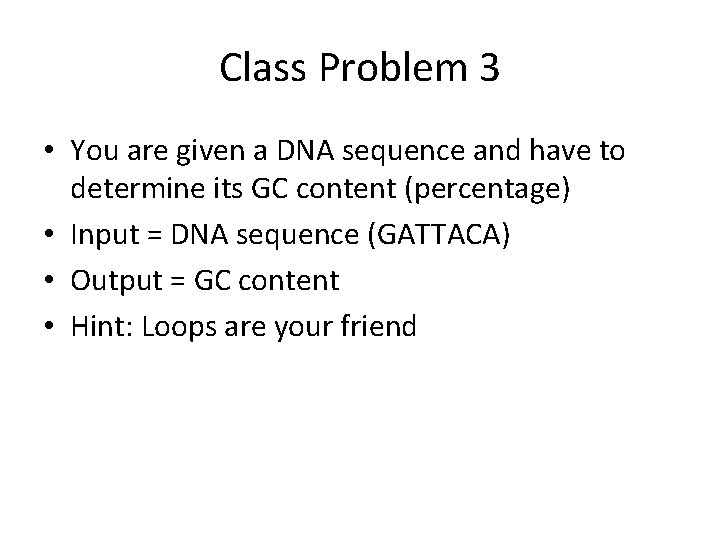Class Problem 3 • You are given a DNA sequence and have to determine its GC content (percentage) • Input = DNA sequence (GATTACA) • Output = GC content • Hint: Loops are your friend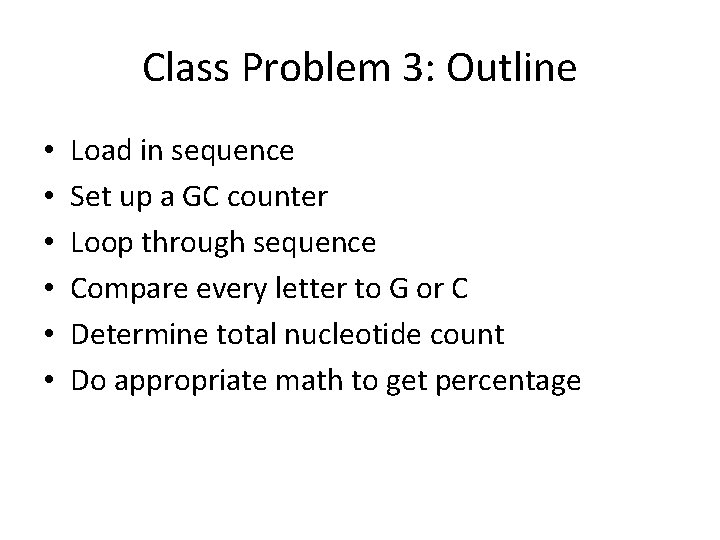Class Problem 3: Outline • • • Load in sequence Set up a GC counter Loop through sequence Compare every letter to G or C Determine total nucleotide count Do appropriate math to get percentageClass Problem 3: Pseudo Code DNASeq = load. File(Input. DNA) GC_Count = 0 For Letter in DNASeq if Letter is G or C GC_Count + 1 DNALength = length(DNASeq) GC = GC_Count/DNALength X 100% Print GCReal Code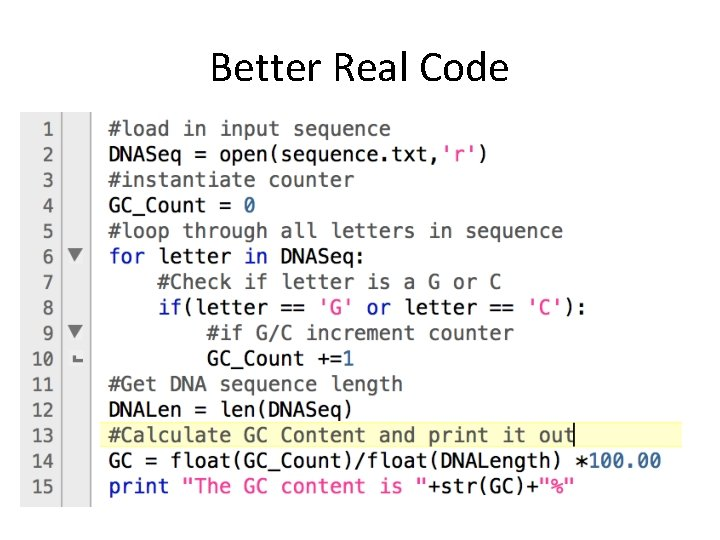Better Real CodeParting Lessons • • • Inputs Outputs Toolbox Take Your Time Only you have to understand your pseudo code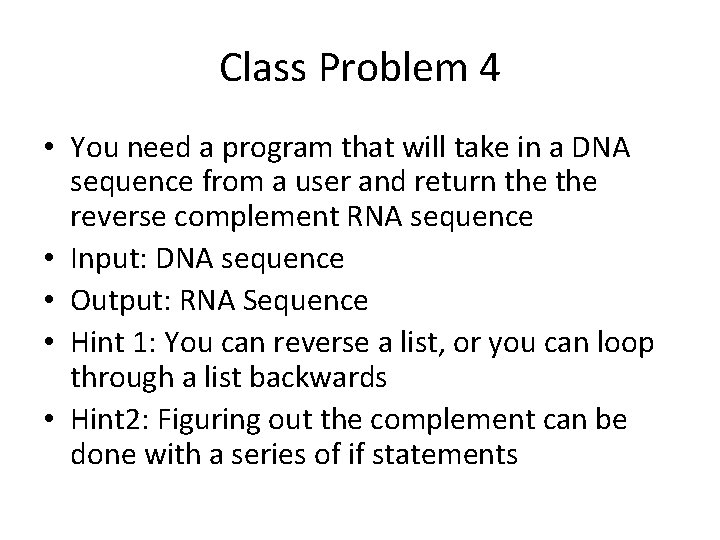Class Problem 4 • You need a program that will take in a DNA sequence from a user and return the reverse complement RNA sequence • Input: DNA sequence • Output: RNA Sequence • Hint 1: You can reverse a list, or you can loop through a list backwards • Hint 2: Figuring out the complement can be done with a series of if statements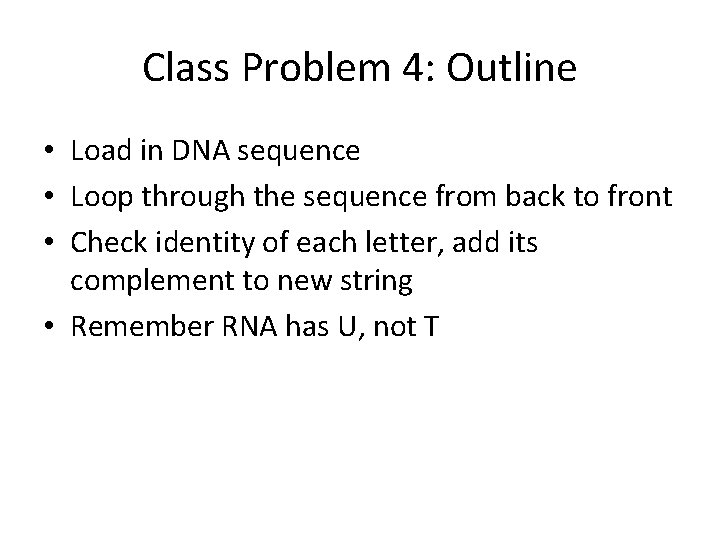Class Problem 4: Outline • Load in DNA sequence • Loop through the sequence from back to front • Check identity of each letter, add its complement to new string • Remember RNA has U, not T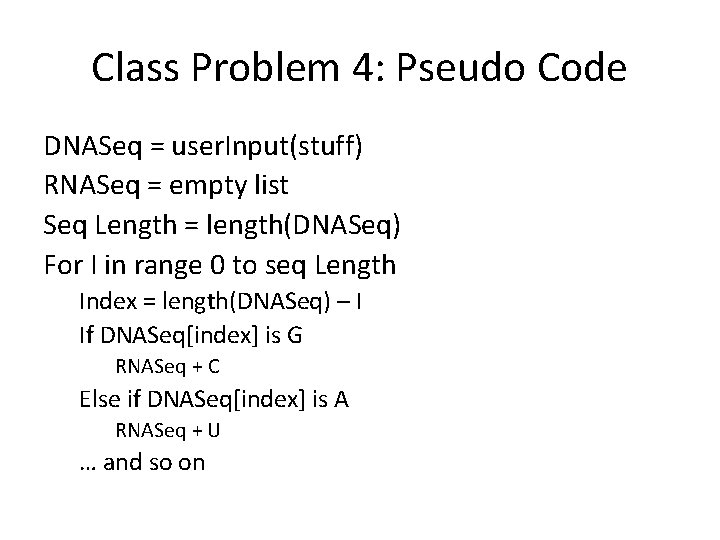Class Problem 4: Pseudo Code DNASeq = user. Input(stuff) RNASeq = empty list Seq Length = length(DNASeq) For I in range 0 to seq Length Index = length(DNASeq) – I If DNASeq[index] is G RNASeq + C Else if DNASeq[index] is A RNASeq + U … and so on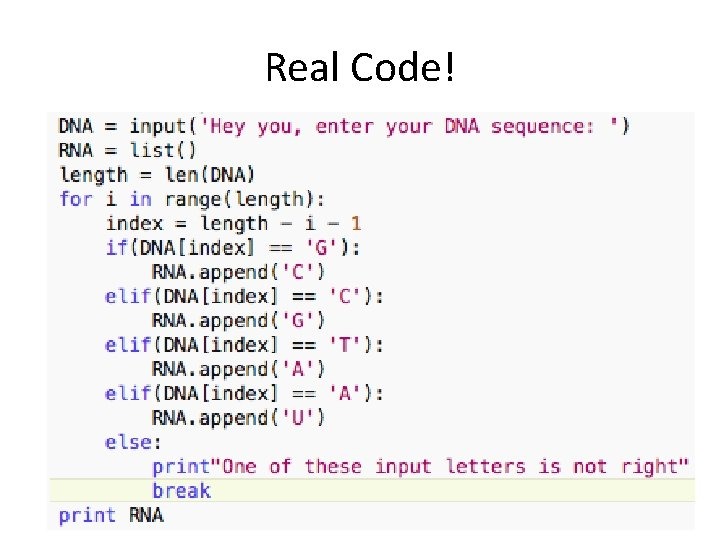Real Code!